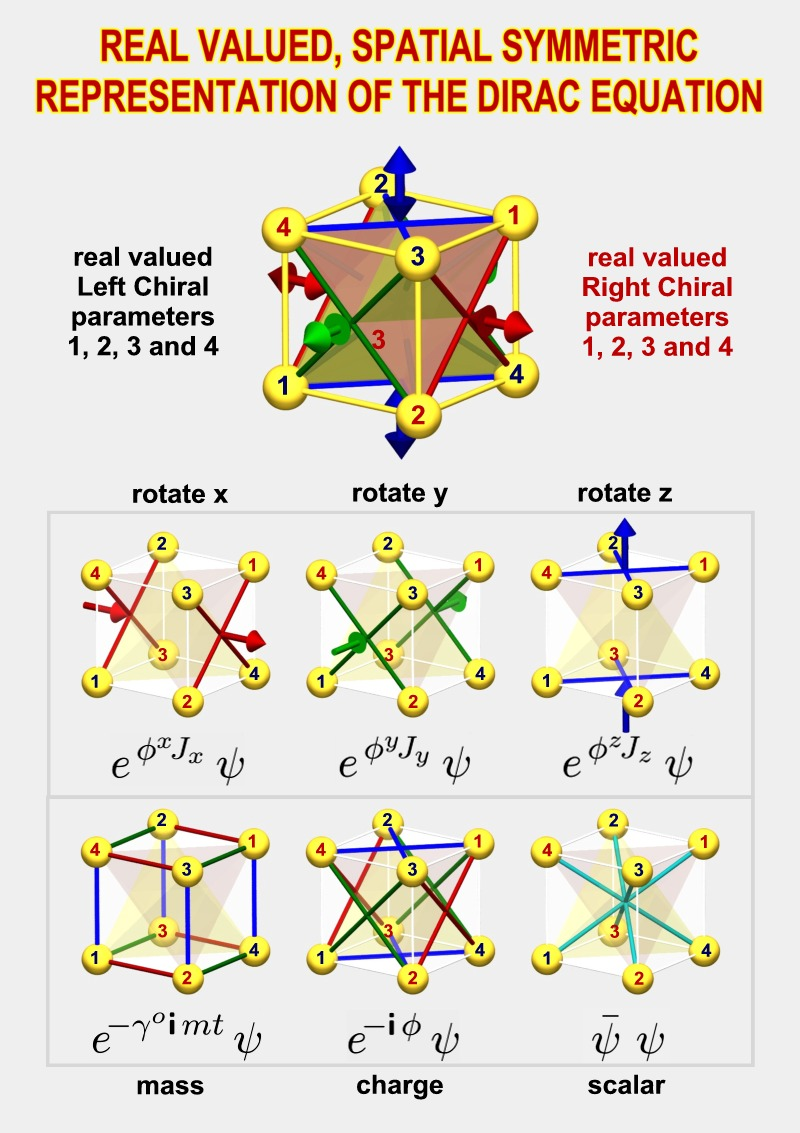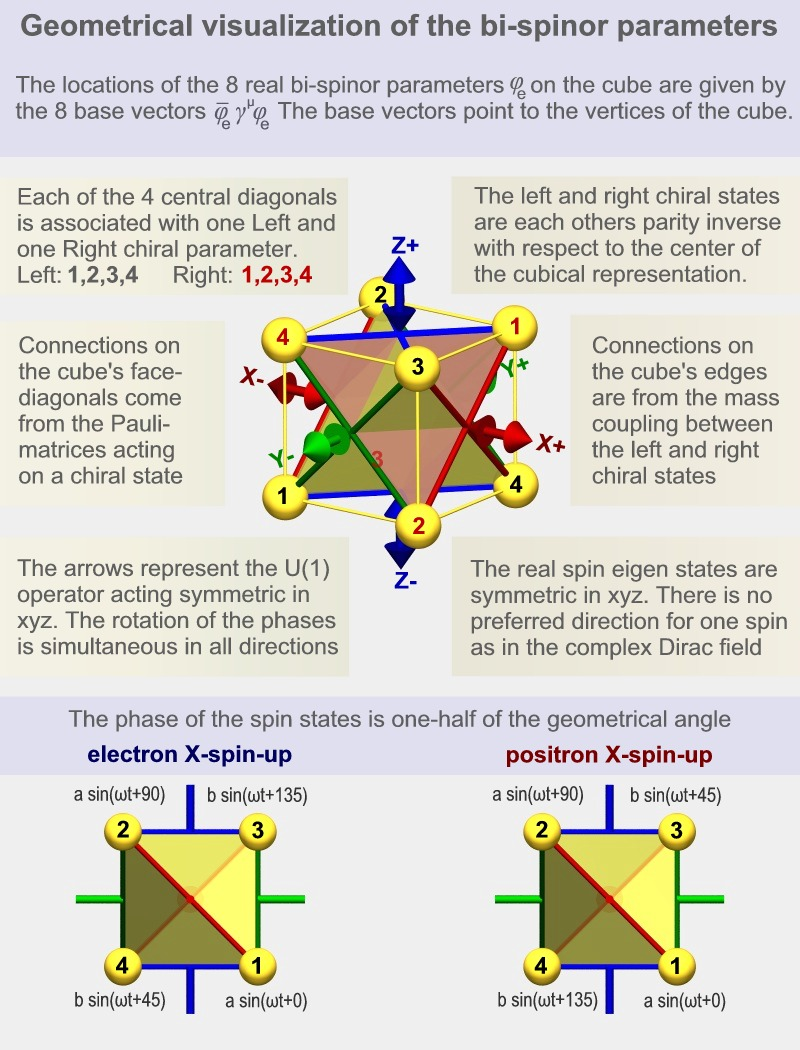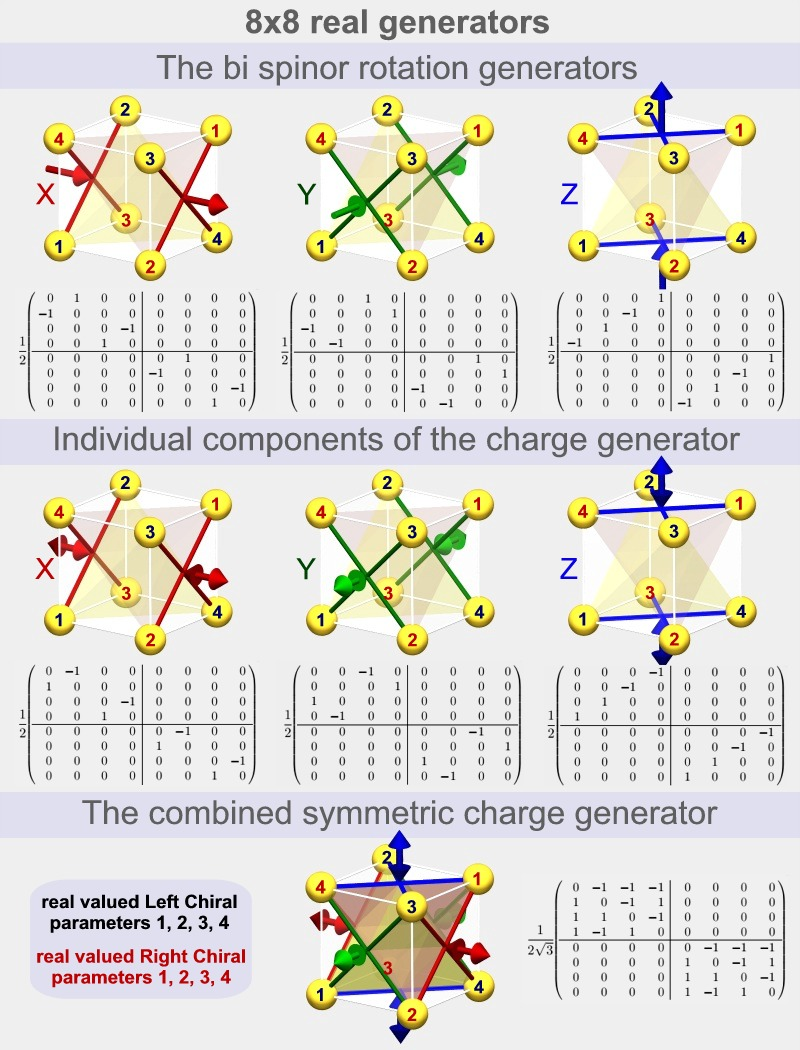20 Sep. 2012

The real, symmetric equation of the electron and its spin.

A mathematical representation that allows a better physical understanding of the electron spin.
by Hans de Vries

The real valued spatially symmetric representation of the Dirac equationThe historical context:  The Schroedinger and Dirac equations.

 The quantum mechanical models of the electron. Since the glorious days of the birth of quantum mechanics in the nineteen twenties humanity knows the elementary "equations of motion"of the electron. The Schroedinger and Dirac equations. Within just a few years after their inception these equations were found to explain Mendeleev's periodic table from 1869 as well as numerous physical experiments. A new era of physics and technical innovation was about to begin. But why are there two different quantum mechanical equations for the electron? Schroedinger equation: Intuitive but far too simple. This equation discovered in 1925 by the Austrian physicist Erwin Schroedinger is the one used by 99% of the engineers, physicist and chemist. It often gives good approximations but has serious short comings: The simplified electron it describes doesn't have a spin. Magnetic properties of materials as we know them don't exist and electrons can freely move at any speed, unrestricted by the speed of light. Gold would have the color of Silver according to Schroedinger's equation, and so on. But it brought us one very important new feature: The interference of matter waves.  The electron field of Schroedinger's equation has 2 values at each point in space, like a complex number. We can separate the two in the amplitude and phase of the field. The phase property of the field is the root cause of matter wave interference. There is something very puzzling with the phase of matter waves here. The familiar physical examples of phase we know arise from rotations or vibrations but If we assume that one of these causes the phase of the matter field then we run into troubles. Rotations and vibrations are always associated with a given direction of the rotation or the vibration. If you add two matter fields then the interference should depend on the "direction" associated with the phase but, it doesn't. This issue is usually swept under the carpet by saying: The matter wave phase stems from a rotation in a so-called isospace.
 Dirac equation: Correct results but undecipherable strange. It was only 3 years, in 1928 that Paul Dirac published his famous paper, "The Quantum theory of the electron" His equation solved all the shortcomings of Schroedinger's equation. He introduced a mathematical model of the electron that essentially survived until the present days. The same model is key, not only in the description of electrons, but also in other particles that were discovered many years later. Most notably the quarks and neutrino's. In the 1950's Dirac's model became the foundation of Quantum Electro Dynamics. The theory capable of predicting a number of physical properties with exceptional precession which are in agreement with experiments with a precision with up to 12 digits. In the 1970's and 1980's this theory was extended to the so called "Standard Model" of physics which included the strong and weak nuclear forces. A real valued and spatially symmetric equation   Unfortunately, in spite of all the success, very few people study the Dirac equation. The combination of its complexity and strangeness provides a barrier for a majority of the students. Most of those that do study the equation and master the required mathematical manipulations are left with an uneasy feeling about the physical meaning of what they are doing. One of the strangest aspects is that the mathematics doesn't treat the x,y and z-axis on equal footing, the representation is not spatially symmetric. While the use of a complex valued field in Schroedinger's equation is bearable. In Dirac's field we deal with 8 values for each point in space either real or imaginary which are very hard to interpret or visualize in a geometric context. What we present in the paper is a 1-to-1 replacement of Dirac's mathematical model which has the same number of parameters (8), produces the same end results. However: all parameters are real valued and the representation is spatially symmetric as one would expect it to be. This model now opens the way for a physical understanding  of  the electron and its spin.

An overview of the real symmetric representation

 The spatial symmetry of the 8 field parameters One major consequence of the spatial symmetry is that the 8 field parameters get a spatial symmetric interpretation as well. The cube as seen in the illustrations occurs if we apply the so called "current" operator to the individual field parameters. Each parameter becomes associated with a propagation (current) from the center of the cube to one of the 8 vertices. Each of the 4 central axis of the cube has two of them, one is left circular polarized and the other is right circular polarized. The eight parameters together define the direction of the electron's current density as well as the direction of the electron's spin. Note that 4 axis-orientations are used to define directions instead of the usual 3 spatial coordinates. This means that there is a one parameter redundancy in the definitions. This redundant parameter turns out to be the phase of the wave function. It's the phase of the wave function which enables the interference of matter waves. We have found a way in which nature can define a phase without needing a special preferred direction of rotation.The 720 degrees of spinor rotation. One of the most puzzling statements a student gets to hear is that "an electron has to rotate over 720 degrees before it returns back to its original position". This statement is directly related to another statement which says that the electron is a spin 1/2 particle. All kinds of strange and often irrelevant visualizations are used by people who are trying to make sense of this. It turns out that we can find the mathematics corresponding to this spin 1/2 behavior directly in the representation itself. We'll see that a more appropriate form of the 720 degrees statement is that "the phase difference is always 1/2 of the geometrical angle" The electron and positron eigenstates. We can calculate the eigenstates of the electron (and the positron) directly from the equation of motion. The particles oscillate with a frequency proportional to their mass. If we look at the parameters along, for instance, the x-axis (in case of a spin in the x direction)  then we see that there are 45 degrees phase relations between parameters that are 90 degrees rotated from each other.   The images above show 4 parameters with phase shifts of 0, 45, 90 and 135 degrees. The other 4 parameters (in the positron case) have phase shifts of 180, 225, 270 and 315 degrees. In the case of the electron there are extra signs. This is what distinguishes the negative charge from the positive charge.

The real symmetric matrices of the representation

 The rotation generators of spin 1/2 particles. We can find the mathematical origin of this spin 1/2 behavior (or 720 degrees behavior) directly in the matrices used in the representation. We can rotate the current and spin direction of the electron field with the use of the x, y and z-rotation matrices shown below. Generally when we perform a continuous rotation then we operate on two (orthogonal) components so that these get a 90 degrees sine-cosine relation. The result is then a circular motion. In the real, symmetric representation however we operate on two parameters which are not orthogonal (90 degrees) but diagonally positioned (180 degrees). The result is that the phase difference is 1/2 of the geometrical angle as is typical for a spin 1/2 particle One can see that each rotation matrix defines four such connections, each time between two parameters. This is why there are four lines shown in each of the images (two X's) The signs in the matrices are so that all four rotations are defined as being in the same direction. The charge generators of spin 1/2 particles. If we place the electron in a potential field then its phase change rate will become higher if the electron's energy becomes higher and lower when the energy becomes lower. If we rotate an electron then we will also change the phase of the parameters (by 1/2 the rate). However if we give the electron a different phase change rate by placing it in a constant potential field then we won't rotate the direction of the spin or the current, now, what is the difference mathematically? We see in the image below that the individual components of the charge generator (which is used to describe the influence of the potential field) are almost identical to the rotation generators. The difference is in the signs.  Two of the four connections are now opposite in sign. The result is that the net rotation is zero. The complete charge generator shown at the bottom is symmetric in x, y and z: The electric potential field has no preferred direction in space.The boost generators of spin 1/2 particles. If we boost a particle then we accelerate the particle to a different speed. The matrices used for this operation are the boost matrices. These matrices are somewhat more complex but from the images we can see that they correspond to the x, y and z-directions. The most important difference with the rotation matrices is that they are non-zero on the diagonals. This causes some parameters to become bigger and bigger when the boost becomes larger, while other parameters become smaller and smaller. the +1's found on the diagonals correspond to the increasing parameters and the -1's correspond to decreasing parameters. The parameters that become bigger are drawn with lighter colors while the ones that become smaller are darker. Now, each parameter corresponds with a current in its direction, from the center of the cube outward. If we boost the electron in a certain direction then we indeed expect the currents in that direction to increase while the currents in the opposite direction should decrease. The mass coupling of the chiral states. If we don't place the electron in a potential field then we still expect that its phase changes continuously and it should do so with a frequency proportional to its mass. The matrix which is responsible for this is the "mass coupling" matrix. This is the matrix which couples the four left chiral parameters with the four right chiral parameters. It is the only matrix which does so. If we compare the 3 individual components of the mass coupling below with the 3 individual components of the charge generator above, then we see that they have the same 4x4 sub matrices but in different quadrants.  This means that the mass coupling has the same effect as the charge generator when the four left chiral parameters are equal to the four right chiral parameters, and the opposite effect if there is a minus sign between the two chiral sets. This is the case in the electron and positron eigenstates respectively. The combined mass coupling matrix is symmetric in x, y and z. Mass does not have a preferred direction in space just like charge doesn't have a preferred direction.The PDF was written over a period of about 3 years. It contains an overview in chapter 1 plus a complete step by step derivation of the real symmetric representation from the standard asymmetric complex one in chapter 2. A lot of attention was spend at the development of the notation. The final form is highly compatible with the standard notation so that most expressions will be either the same or almost identical.The reader will be able to switch between the representations without effort. The real symmetric representaion is obtained by going from the standard complex 2x2 Pauli matrices to their equivalent 4x4 real versions. The extra degrees of freedom obtained make it possible to make the representation symmetric. The consequence of the 4x4 matrices is that SO(4) becomes the group of unitary generators of rotation and charge. The non-Abelian charges introduced are discussed in chapter 3. Further chapters are under development.